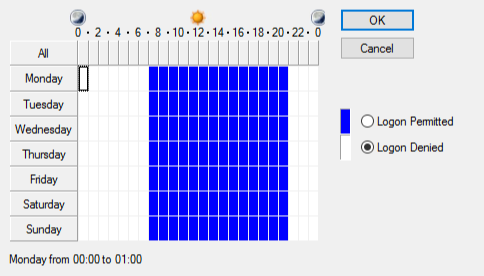# Log On hours with powershell

We had a requirement recently to set logon hours for a specific set of users. To achieve this, we set up one user through the AD User and computers snap-in:We then created a script (borrowing from other snippets/scripts we found on the web) that would interrogate the source users logon hours, and then allow us to apply them to all users in a group:
``````#############################################################
#         Simple script to get and set logon hours          #
#                                                           #
# Set up an account through ADUC and configure log on hours #
#                                                           #
#    This script will then enumerate this and set hours     #
#                                                           #
#                 on the group you specify                  #
#############################################################
\$validuser = \$false
\$validgroup = \$false

while(!(\$validuser)){
clear-host
write-host "
__                 ___
/ /  ___   __ _    /___\_ __     /\  /\___  _   _ _ __ ___
/ /  / _ \ / _  |  //  // '_ \   / /_/ / _ \| | | | '__/ __|
/ /__| (_) | (_| | / \_//| | | | / __  / (_) | |_| | |  \__ \
\____/\___/ \__, | \___/ |_| |_| \/ /_/ \___/ \__,_|_|  |___/
|___/
" -ForegroundColor Cyan
\$user = read-host Enter the user name of the log on hours you wish to duplicate:
\$testresult = ([ADSISearcher] "(sAMAccountName=\$user)").FindOne()
If(\$null -ne \$testresult){
\$validuser = \$true
}Else{
Write-host "No such user!" -foregroundcolor red
start-sleep -seconds 2
}
}
write-Host Logon Hours for this user are: -ForegroundColor Green
\$logonhours = get-aduser \$user -property logonhours | select-object -expand logonhours
\$dn = (get-aduser \$user -property distinguishedname | select-object distinguishedname).distinguishedname
[byte[]]\$hours1 = \$logonhours

\$Bias = [Math]::Round((Get-ItemProperty `
-Path HKLM:\System\CurrentControlSet\Control\TimeZoneInformation).Bias/60, `
0, [MidpointRounding]::AwayFromZero)

# Create an empty array with 168 elements, one for each hour of the week.
\$LH = New-Object 'object[]' 168

Function OctetToHours (\$Octet)
{
# Function to convert Octet value (byte array) into binary string
# representing the logonHours attribute. The 168 bits represent 24 hours
# per day for 7 days, Sunday through Saturday. The logonHours attribute
# in Active Directory is in UTC. This function converts into the local
# time zone. If the bit is "1", the user is allowed to logon during
# that hour. If the bit is "0", the user is not allowed to logon.

If (\$Octet -eq \$Null)
{
# If the logonHours attribute has no value, the user is allowed
# logon all hours.
For (\$j = 0; \$j -le 167; \$j = \$j + 1)
{
\$LH[\$j] = "1"
}
}
Else
{
# Loop through the 21 bytes in the array, each representing 8 hours.
For (\$j = 0; \$j -le 20; \$j = \$j + 1)
{
# Check each of the 8 bits in each byte.
For (\$k = 7; \$k -ge 0; \$k = \$k - 1)
{
# Adjust the index into an array of hours for the
# local time zone bias.
\$m = 8*\$j + \$k - \$Bias
# The index into the  array of hours ranges from 0 to 167.
If (\$m -lt 0) {\$m = \$m + 168}
# Check the bit of the byte and assign the corresponding
# element of the array.
If (\$Octet[\$j] -band [Math]::Pow(2, \$k)) {\$LH[\$m] = "1"}
Else {\$LH[\$m] = "0"}
}
}
}

"Day"
"of   ------- Hour of the Day -------"
"Week M-3 3-6 6-9 9-N N-3 3-6 6-9 9-M"
\$arrDays = ("Sun", "Mon", "Tue", "Wed", "thu", "Fri", "Sat")

# Loop through the array of 168 hours.
For (\$j = 0; \$j -le 167; \$j = \$j + 1)
{
If ((\$j % 24) -eq 0)
{
# First hour of a day.
\$Line = \$arrDays[\$j / 24] + " "
}
If ((\$j %3) -eq 0)
{
# Insert a space every 3 hours for legibility.
\$Line = \$Line + " "
}
\$Line = \$Line + \$LH[\$j]
# If this is the last hour of the day, output.
If (((\$j + 1) % 24) -eq 0) {\$Line}
}
}

"User: " + \$User

OctetToHours \$mUser.logonHours
\$check = Read-host "Is this correct? (y/n)"
if(\$check -eq "n"){
write-host Please reconfigure the source account correctly and try again -ForegroundColor Red
Exit
}

while(!(\$validgroup)){
clear-host
write-host "
__                 ___
/ /  ___   __ _    /___\_ __     /\  /\___  _   _ _ __ ___
/ /  / _ \ / _  |  //  // '_ \   / /_/ / _ \| | | | '__/ __|
/ /__| (_) | (_| | / \_//| | | | / __  / (_) | |_| | |  \__ \
\____/\___/ \__, | \___/ |_| |_| \/ /_/ \___/ \__,_|_|  |___/
|___/
" -ForegroundColor Cyan
write-host Source Account is \$user -ForegroundColor Green
\$group = read-host Enter the name of the group you wish to apply this to:

\$GroupExists = Get-ADGroup -LDAPFilter "(SAMAccountName=\$group)" -ErrorAction SilentlyContinue
If(!\$GroupExists){
Write-host "No such group!" -foregroundcolor red
start-sleep -seconds 2
}Else{
write-host Group Exists!
\$validgroup = \$true
}
}
#ok we have a valid group, lets proceed...
\$members = Get-ADGroupMember -Identity \$group -Recursive | Select -ExpandProperty samAccountName

# Iterate through each member of the current group
ForEach (\$member in \$members) {
Get-ADUser -Identity \$member |
Set-ADUser -Replace @{logonhours = \$hours1}
Write-Host "Setting GROUP 1 logon times for \$member, a member of group \$group."
}

write-host "All Done!" -ForegroundColor Green
``````

Simply run the script, input the username of the user you have already configured logon hours for, then when prompted, enter the group name you wish to apply the logon hours to.

Enjoy!

This site uses Akismet to reduce spam. Learn how your comment data is processed.